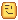# Getting's players position on an axis

Hello random strangerI want to get player’s position relative to a brick’s X and Z axis.

In other words, what I mean; I need to place an attachment, I’m gonna parent it to that brick. Since WorldPosition doesn’t work like I imagined it would, i need to calculate player’s character position relative to the part. (I wanna fill in the `Position` property of an attachment.)

# Solution will be marked.

If I didn’t explain something clearly here or made an error, please comment down below, i’ll be quick to reply.

All feedback is helpful!``````local pos = player.Character.HumanoidRootPart.Position

local x_axis = pos.X
local z_axis = pos.Z

print('Z: '..x_axis)
print('X: '..z_axis)``````

That’s not what I meant, let me clarify - I dont need the player’s world axis positon; I need their position on the brick’s axis, where the center of the brick represents 0.

Oh

``````local x_axis = (pos-  brick.Position).X
local z_axis = (pos - brick.Position).Z
``````

Z and X relative to the brick.Position.

Or just put the brick at `0,0,0`

Either use

``````local relativeCFrame = CFrame1:ToWorldSpace(CFrame2)
``````

or

``````local relativeCFrame = CFrame1:ToObjectSpace(CFrame2)
``````

Note that they’re basically the same, because the following is true:

``````CFrame1:ToWorldSpace(CFrame2) == CFrame2:ToObjectSpace(CFrame1)
``````

The 2 CFrames you’d need is the brick’s & Player’s Root
Then you use the following:

``````print(relativeCFrame.X, relativeCFrame.Y, relativeCFrame.Z)
``````

This should work too if you would rotate the part around!

Thank you kind stranger! I’ll try it out, if it solves my issue i’ll mark your comment as the solution or otherwise i’ll follow up.